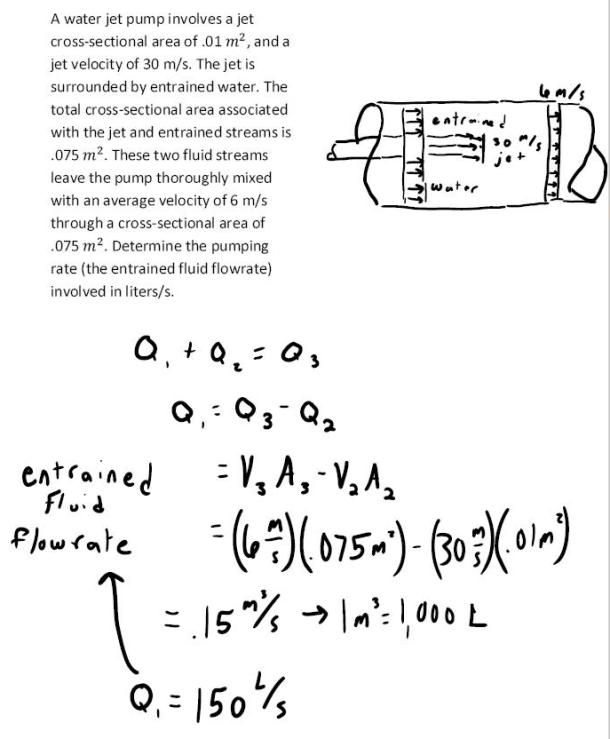fluid mechanics math problems engineering equations fluid mechanics basic mechanics school homework engineering math fluids formulas fluid mechanics problems fluid mechanics problem solutions to fluids problems full solution fluid mechanics engineering fluids problem solution fluid mechanics math problems engineering equations fluid mechanics basic mechanics school homework engineering math fluid mechanics formulas fluid problems fluid mechanics problem solutions to fluid mechanics problems full solution fluid mechanics engineering fluid mechanics problem solution
fluid mechanics math problems engineering equations fluid mechanics basic mechanics school homework engineering math fluids formulas fluid mechanics problems fluid mechanics problem solutions to fluids problems full solution fluid mechanics engineering fluids problem solution fluid mechanics math problems engineering equations fluid mechanics basic mechanics school homework engineering math fluid mechanics formulas fluid problems fluid mechanics problem solutions to fluid mechanics problems full solution fluid mechanics engineering fluid mechanics problem solution
Highalphabet Home Page fluid mechanics solutions fluids math solved Fluid Mechanics Page 1
A water jet pump involves a jet cross-sectional area of .01 m^2, and a jet velocity of 30 m/s. The jet is surroundd by entrained water. the total cross-sectional area associated with the jet and entrained streams is .075 m^2. These two fluid streams leave the pump thoroughly mixed with an average velocity of 6 m/s through a cross-sectional area of .075 m^2. Determine the pumping rate (the entrained fluid flowrate) involved in liters/s.A water jet pump involves a jet cross-sectional area of .01 m^2, and a jet velocity of 30 m/s. The jet is surroundd by entrained water. the total cross-sectional area associated with the jet and entrained streams is .075 m^2. These two fluid streams leave the pump thoroughly mixed with an average velocity of 6 m/s through a cross-sectional area of .075 m^2. Determine the pumping rate (the entrained fluid flowrate) involved in liters/s.